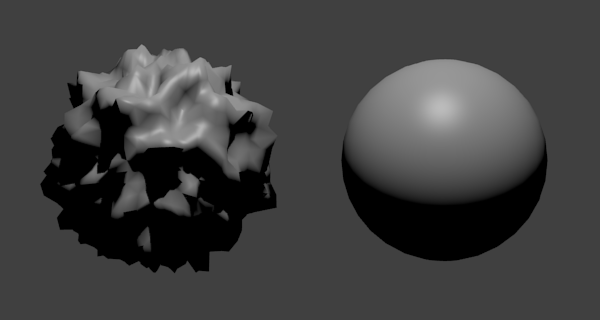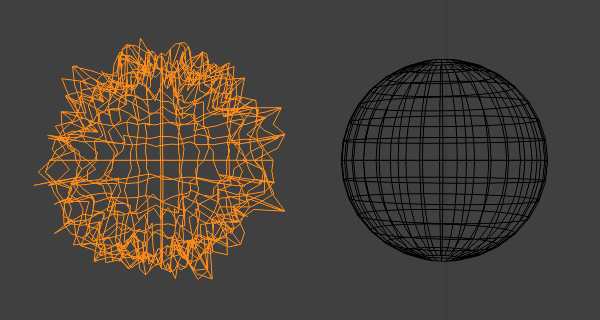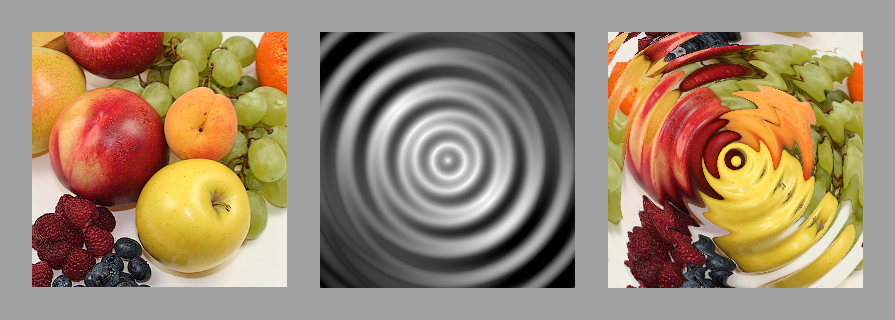io7m | single-page | multi-page | archive (zip, signature)
 1. Overview 2D GPU displacement mapping 3. Procedural textures Previous Up Next

Examples
3D vertex displacement
3D vertex displacement is used by artists to produce extremely complex models using only simple meshes and textures.In the above image, each vertex is translated along its own normal vector by a value K, where K is obtained by:
1. Look up the pixel P at coordinates (s, t) in an associated greyscale texture.
2. Interpret P as a value in the range -0.5 .. 0.5 inclusive.
3. (Optionally) Multiply P by some configurable maximum M.
The actual (s, t) coordinates used can be picked at random or, more commonly, can simply be the texture coordinates associated with each vertex in the mesh as is normal with any textured model. Higher values of M result in more pronounced displacement.
The displacement map/texture used in the depicted example is a randomly generated greyscale noise texture.
2D pixel displacement
2D pixel displacement can be used to produce complex procedural textures from simple components. A rippling "liquid" effect can be produced by displacing each pixel in a texture by another generated texture:In the above image, each pixel is translated up and left by K pixels where K is obtained by:
1. Look up the pixel P at coordinates (s, t) in an associated greyscale texture.
2. Interpret P as a value in the range 0.0 .. 1.0 inclusive.
3. Multiply P by 20.
The actual (s, t) coordinates used are the (x, y) coordinates of the corresponding pixel in the image being displaced.
Displacement, then, isn't the act of modifying pixels, but the act of modifying the coordinates that would have been used to select pixels.

 Previous Up Next 1. Overview 2D GPU displacement mapping 3. Procedural textures